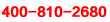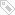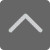# 关于小学一年级口算练习题

2019-01-25 11:59:01 　来源：奥数网

关于小学一年级口算练习题！同学们我们知道，数学是跟生活息息相关的。数学提高的是逻辑计算能力，这些在生活中都要不断的用到。下面就是小编为大家带来的关于小学一年级口算练习题，希望可以帮助到大家。

想要了解小学一年级练习题的相关资料，请点击加入【爱智康小学交流福利群】，并直接向管理员“小康康”索取!爱智康小学交流福利群会不定期免费发放学习资料，小学政策等相关消息，请持续关注!

41) 13+7= 42) 7+13= 43) 1+12= 44) 1+14= 45) 11+9=

46) 12+0= 47) 13+2= 48) 10+0= 49) 3+13= 50) 2+14=

51) 1+18= 52) 16+2= 53) 11+2= 54) 3+10= 55) 17+2=

56) 10+4= 57) 13+1= 58) 8+12= 59) 17+3= 60) 0+17=

小编推荐：

以上就是小编特意为大家整理的关于小学一年级口算练习题希望对有需要的同学提供帮助，大家如果在学习中有什么疑问，欢迎拨打爱智康免费电话：!那里有专业的老师为大家解答。

### 2019年精品学习包：

•### 小学语|数|英

历年试卷、北京30所校练习题、名著|重点校巡礼|等100讲视频、纸质书籍领取

•### 初中九大学科

历年真题、期末|期中|志愿填报|重点校巡礼|等100讲视频、纸质书籍领取

•### 高中九大学科

历年真题、期末|期中|志愿填报|重点校巡礼|等100讲视频、纸质书籍领取

•### 更新中

学习包内容持续更新中......标签：　关于小学一年级口算练习题• 全国课程在线咨询
• 咨询热线：4000-121-121
• 扫描注册有礼

• 让学习更有效